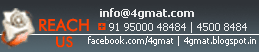Home GMAT Classes GMAT eBooks Math Books Practice Test Forums B Schools About Us

## Quiz 17 : Question 3 of 10

If 'a' and 'b' are the roots of the quadratic equation x2 - x + 1 = 0, what is the value of a2 + b2?

A.     1

B.     0

C.     -1

D.     2

A.     -2